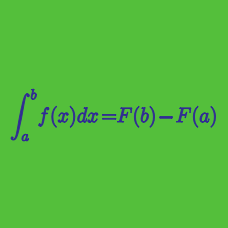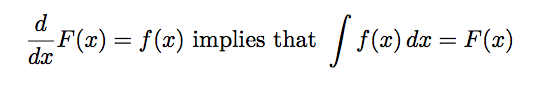Calculus

# Fundamental Theorem of Calculus

Evaluate the definite integral $\int_{0}^{2}\left( \sin^2 x-x \right)dx - \int_{2}^{0}\left( \cos^2 x-x \right)dx.$

If $\displaystyle f(x) = \int_{x}^{x+1} (2t^2+t)\ dt$, what is the value of $f'(7)$?

The volume of water in a container at a height of $x\ \mbox{cm}$ from the bottom, can be expressed as $V(x) = x^3-3x^2+4x$ in $\mbox{cm}^3$. If the surface area of water at heights of $y \ \mbox{cm}$ and $\frac{1}{6}y \ \mbox{cm}$ are the same, then $y=\frac{a}{b}$, where $a$ and $b$ are coprime positive integers. What is the value of $a+b$?Is the above statement true?

Given function $g(x)=\int_{0}^{x} \sqrt{1+t^{3}}dt,$ what is $g'(x)?$

×